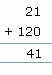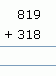Name: ___________________Date:___________________

 Email us to get an instant 20% discount on highly effective K-12 Math & English kwizNET Programs!

### Grade 3 - Mathematics5.10 Addition with Regrouping

1. Start from the ones place
2. Add the numbers in the ones place.
3. Add the numbers in the tens place.
4. Add the numbers in the hundreds place.
 Question Step 1 Step 2 Step 31. Start from the ones place, add the numbers in that place.
If the sum is one digit, place in the units place.
If the sum is two digits, place right number in the units place and left number as carryover.
2. Add the numbers in the tens place (also add carryover if any)
If the sum is one digit, place in the units place.
If the sum is two digits, place right number in the units place and left number as carryover.
3. Add the numbers in the hundreds place (also add carryover if any)
If the sum is one digit, place in the units place.
If the sum is two digits, place right number in the units place and left number as carryover.

 Question Step 1 Step 2 Step 3Add units digits Add tens digits and carryover Add hundreds digits 9+8=17 1+1+1=3 8+3=11

Start from the ones place, add the numbers in that place, then move on to the tens and to the hundreds.
Here we get 9+8 = 17, since it a 2 digit number we write 7 under ones place and take 1 as carry over in the tens place.
Hence in tens place we have 1+1+1 = 3, since it is a 1 digit number, write 3 under the tens place.
In hundreds place we get, 8+3 = 11. Hence the answer is 1137
Directions: Find the sum of the 3 digit numbers. Also write at least ten examples of your own of each kind.Name: ___________________Date:___________________

### Grade 3 - Mathematics5.10 Addition with Regrouping

 Q 1: 846 + 318 =1164164016802490 Q 2: 632 + 848 =1480124016202400 Q 3: 357 + 951 =102013082001200 Q 4: 748 + 282 =1039100010321030 Question 5: This question is available to subscribers only! Question 6: This question is available to subscribers only!

#### Subscription to kwizNET Learning System costs less than \$1 per month & offers the following benefits:

• Unrestricted access to grade appropriate lessons, quizzes, & printable worksheets
• Instant scoring of online quizzes
• Progress tracking and award certificates to keep your student motivated
• Unlimited practice with auto-generated 'WIZ MATH' quizzes